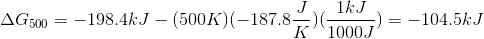# AP Chemistry : Thermodynamics

## Example Questions

### Example Question #4 : Gibbs Free Energy And Spontaneity

A system with __________ and __________ will never be spontaneous.

positive enthalpy . . . negative entropy

positive enthalpy . . . positive entropy

negative enthalpy . . . positive entropy

negative enthalpy . . . negative entropy

positive enthalpy . . . negative entropy

Explanation:

The Gibb's free energy equation is used to determine the spontaneity of a reaction and is written as follows:.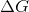is Gibb's free energy,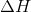is enthalpy, and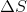is entropy. In order for a reaction to be spontaneous, Gibb's free energy must have a negative value. Based on the equation, we can see that a positive enthalpy in combination with a negative entropy will always result in a positive value for Gibb's free energy.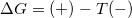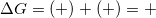This means these are the conditions that will always result in a nonspontaneous reaction.

### Example Question #5 : Gibbs Free Energy And Spontaneity

Consider the following reaction in a galvanic cell: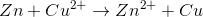Which if the following statements about the reaction is false?

The cell potential is positive

The reaction is spontaneous

The equilibrium constant is greater than one

Gibb's free energy is positive

Gibb's free energy is positive

Explanation:

A galvanic cell results in a positive cell potential from a spontaneous reaction. Spontaneous reactions always have a negative Gibb's free energy.

An equilibrium constant greater than one would indicate that the equilibrium concentration of products is greater than the equilibrium concentration of reactants, consistent with a spontaneous reaction.

### Example Question #6 : Gibbs Free Energy And Spontaneity

Consider the following reaction in a galvanic cell: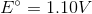What is the Gibb's free energy for the reaction under standard conditions?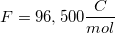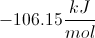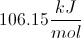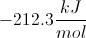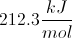Explanation:

You can find the Gibb's free energy of a galvanic cell by using the following equation: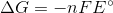is the number of moles of electrons that are transferred in the reaction,is Faraday's constant, and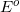is the potential of the cell.

We are given the constant value and the cell potential. The moles of electrons transferred is equal to the change in charge on the atoms in the reaction. In this reaction, copper is reduced from a charge of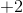to zero, requiring that is gained two moles of electrons.

Using these values, we can find Gibb's free energy for the cell.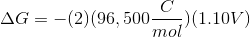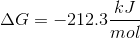Notice that the value is negative. Galvanic cells are spontaneous, and will have negative Gibb's free energies at standard conditions.

### Example Question #7 : Gibbs Free Energy And Spontaneity

If the reaction quotient (Q) is less than the equilibrium constant (K), what is true about the Gibbs free energy?

It is greater than zero

It is equal to zero

We must know the reaction enthalpy to determine the answer

We must know the reaction temperature to determine the answer

It is less than zero

It is less than zero

Explanation:

If Q is less than K, then the reaction has not yet reached the equilibrium state. It will proceed spontaneously in the forward direction. Since it is proceeding spontaneously in the forward direction, this must mean that the ΔG (Gibbs free energy) must be negative, or less than zero.

Enthalpy and temperature are necessary when mathematically evaluating Gibbs free energy, but the reaction quotient and equilibrium constant provide enough information to qualitatively determine that the reaction is proceeding spontaneously.

### Example Question #8 : Gibbs Free Energy And Spontaneity

Of the following reactions, which of the following is only spontaneous at high enough temperatures?

∆H +, ∆S –

∆H +, ∆S +

None of the Above

∆H –, ∆S –

∆H –, ∆S +

∆H +, ∆S +

Explanation:

Based on Gibbs Free Energy, ∆G = ∆H – T∆S, we find that ∆H– will contribute to spontaneity (∆G<0). Since we are looking at temperatures, in order for ∆S to make an nonspontaneous reaction spontaneous at only high temperatures, ∆H will be positive, and ∆S will be positive.

### Example Question #9 : Gibbs Free Energy And Spontaneity

If a reaction has a positive value for its enthalpy and a negative value for its entropy, which of the following is true?

The reaction is spontaneous
Not enough information to tell
The reaction is nonspontaneous
The reaction is at equilibrium
Correct answer: The reaction is nonspontaneous
Explanation:

Given the equation for free energy, (delta)G = (delta)H-T(delta)S, we can determine that the reaction is nonspontaneous at all temperatures if H is positive and S is negative. This combination would always lead to a positive G value, meaning that free energy is required for the reaction to take place and it is therefore nonspontaneous.

### Example Question #10 : Gibbs Free Energy And Spontaneity

What must be true of a spontaneous process?

ΔG → 0 and K → Q

K ⇌ 0

ΔG ← 0 and K → Q

ΔG ← 0 and K ← Q

ΔG → 0 and K ← Q

ΔG ← 0 and K → Q

Explanation:

Change in free energy must always be negative for a spontaneous process. Additionally, Q must be less than K so that the reaction will proceed in the forward reaction, toward equilibrium.

### Example Question #91 : Thermochemistry And Kinetics

Na(s) + 1/2 Cl2(g) → NaCl (s)

The Standard Heat of formation for NaCl is -411.1 kJ

Which of the following descriptions accurately describes the reaction above?

It is favorable and driven by a change in entropy only

It is favorable and driven by change in enthalpy only

It is unfavorable and it is hindered by the unfavorable change in enthalpy

It is favorable and driven by a change in enthalpy and entropy

It is favorable and driven by change in enthalpy only

Explanation:

A reaction being favorable or unfavorable is largely determined by the thermochemistry of the reaction.  More specifically it is determined by the change in gibbs free energy which is determined by the change in enthalpy, the change in entropy, and the temperature of the reaction.  Here we are told that the reaction has a favorable enthalpy change (- means energy is released).  Qualitatively we can see that the reaction will have an unfavorable change in entropy because the product, being a solid, is more ordered than the reactants which are both solid and gaseous.  Thus we can conclude that the reaction is favorable because of the favorable change in enthalpy, which helps to overcome the unfavorable change in entropy.

### Example Question #561 : Ap Chemistry

Calcium carbonate is formed from calcium oxide and carbon dioxide.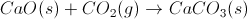Given that this reaction is spontaneous at low temperatures and non-spontaneous at high temperatures, what must be true about the change of enthalphy () and the change of entropy ()?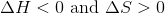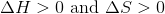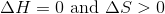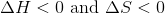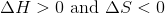Explanation:

A reaction is spontaneous if the change of Gibb's free energy (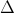G) is less than zero. Recall that (G) is related toH andS by the equation below.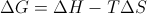For this particular reaction,S is negative because the total number of moles of gas decreases from reactants to products. Since the reaction is spontaneous at lower temperatures, thenG must be negative when T is small. Since the -TS term would be positive for all values of T, the only wayG can be negative is ifH is negative. At higher temperatures, the positve -TS term would outweigh the negativeH term, resulting in a positiveG and a non-spontaneous reaction.

### Example Question #51 : Thermodynamics

Consider the following reaction: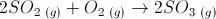At 298K,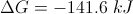,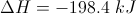, and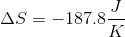. Assuming that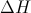and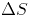do not change with temperature, what is the value of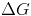at 500K? Does the reaction become more or less spontaneous?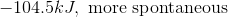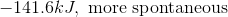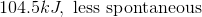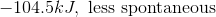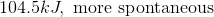We know that, so